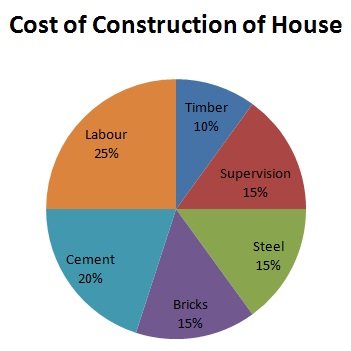# Statistics - Pie Chart

A pie chart (or a pie graph) is a circular statistical graphical chart, which is divided into slices in order to explain or illustrate numerical proportions. In a pie chart, centeral angle, area and an arc length of each slice is proportional to the quantity or percentages it represents. Total percentages should be 100 and total of the arc measures should be 360° Following illustration of pie graph depicts the cost of construction of a house.From this graph, one can compare the sum spent on cement, steel and so on. One can also compute the actual sum spent on each individual expense. Consider an example, where we want to know how much more is the labour cost when compared to cost of steel.

${ Amount\ spent\ on\ labor\ = \frac{90}{60} \times 600000 = \$\ 150000 \\[7pt] \ Sum\ spent\ on\ steel\ = \frac{54}{360} \times 600000 = \$\ 90000 \\[7pt] \ Excess = 150000 - 90000 = \$\ 60000 \\[7pt] \ Let\ 60000=x\% \ of\ 600000. \\[7pt] \implies \frac{x}{100} \times 600000 = \$\ 60000. \\[7pt] \implies x = 10\% \ of\ total\ expense. }$